## Contour Integration

Let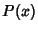and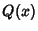be Polynomials of Degreesandwith Coefficients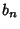, ...,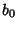and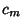, ...,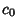. Take the contour in the upper half-plane, replaceby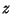, and write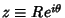. Then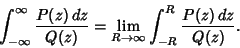(1)

Define a path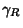which is straight along the Real axis from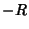to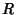and makes a circular arc to connect the two ends in the upper half of the Complex Plane. The Residue Theorem then gives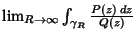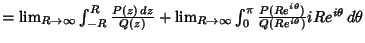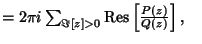(2)
where Res denotes the Residues. Solving,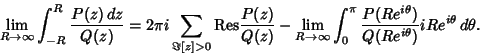Define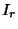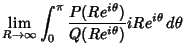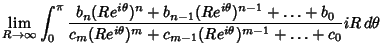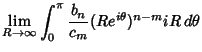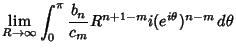(3)

and set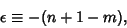(4)

then equation (3) becomes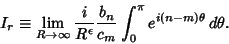(5)

Now,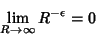(6)

for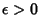. That means that for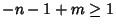, or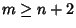,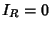, so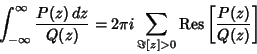(7)

for. Apply Jordan's Lemma with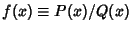. We must have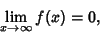(8)

so we require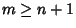. Then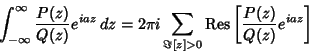(9)

for.

Since this must hold separately for Real and Imaginary Parts, this result can be extended to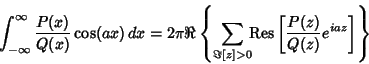(10)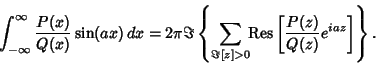(11)

It is also true that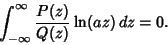(12)

See also Cauchy Integral Formula, Cauchy Integral Theorem, Inside-Outside Theorem, Jordan's Lemma, Residue (Complex Analysis), Sine Integral

References

Morse, P. M. and Feshbach, H. Methods of Theoretical Physics, Part I. New York: McGraw-Hill, pp. 353-356, 1953.